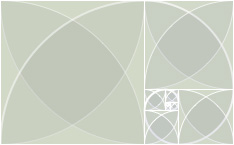# Fibonacci Spiral

Posted on in Code Sketches

I am fascinated by the Fibonacci (Golden) Spiral, and love the geometric construction, which is mimicked and expanded in this Processing sketch.Fibonacci Spiral by Nathan Selikoff. 2010. Image capture from Processing sketch.

While developing an earlier concept for my website redesign, I created this version of the Fibonacci Spiral, with subtle coloration and fading arcs and squares.

``````// large version
// second attempt at a fibonacci spiral
// first attempt was great, but used rotation,
// which is expensive and imprecise
// here's the beginning of the fibonacci sequence...
// 0, 1, 1, 2, 3, 5, 8

void setup() {
size(611, 378);
smooth();
frameRate(30);
}

int[] s = new int;
int tmp, phase;
float speed;

void draw() {
// clear the screen to a nice green
background(228, 236, 215);

// initialize variables
s = 0;
s = 0; // first number of the fibonacci sequence
s = 1; // second number of the fibonacci sequence
phase = 0; // simple counter; use this to apply fx to the boxes linearly
speed = 0.01; // speed of the effects

// set the origin at the end of the spiral
translate(442, 272);

// flip vertically to match my logo
scale(1.0, -1.0);

rotate(PI);

// this loop constructs the spiral
// basically, draw a square of side s, rotate 90deg, calculate the new s0 and s,
// move up s0 + s, and repeat...
while (s < 611) {

// use a sin function to give us some nice undulating effects
float wave = sin((frameCount + phase*20) * speed) + 1.0;

// styles for the arcs
fill(0, 0, wave * 64, 10); // undulating transparent black fill
stroke(255, wave * 255); // undulating white stroke

// draw the quarter-circles inside the squares
// set the stroke weight to 2 for the arc that makes the spiral
if (phase % 4 == 0) strokeWeight(2); else strokeWeight(1);
arc(s, s, 2*s, 2*s, PI, 3*PI/2.0);
if (phase % 4 == 1) strokeWeight(2); else strokeWeight(1);
arc(0, s, 2*s, 2*s, -PI/2.0, 0);
if (phase % 4 == 2) strokeWeight(2); else strokeWeight(1);
arc(0, 0, 2*s, 2*s, 0, PI/2.0);
if (phase % 4 == 3) strokeWeight(2); else strokeWeight(1);
arc(s, 0, 2*s, 2*s, PI/2.0, PI);

// styles for the squares
noFill();
stroke(255, 255, 255, 255);
strokeWeight(1);

// draw the square
rect(0, 0, s, s);

// calculate the next number in the fibonacci sequence
tmp = s;
s += s;
s = s;
s = tmp;

// translate to get in position for the next square
switch(phase % 4) {
case 0:
translate(s, 0);
break;
case 1:
translate(-s, s);
break;
case 2:
translate(-s, -s);
break;
case 3:
translate(0, -s);
break;
}

phase++;
}
}
``````

Φ